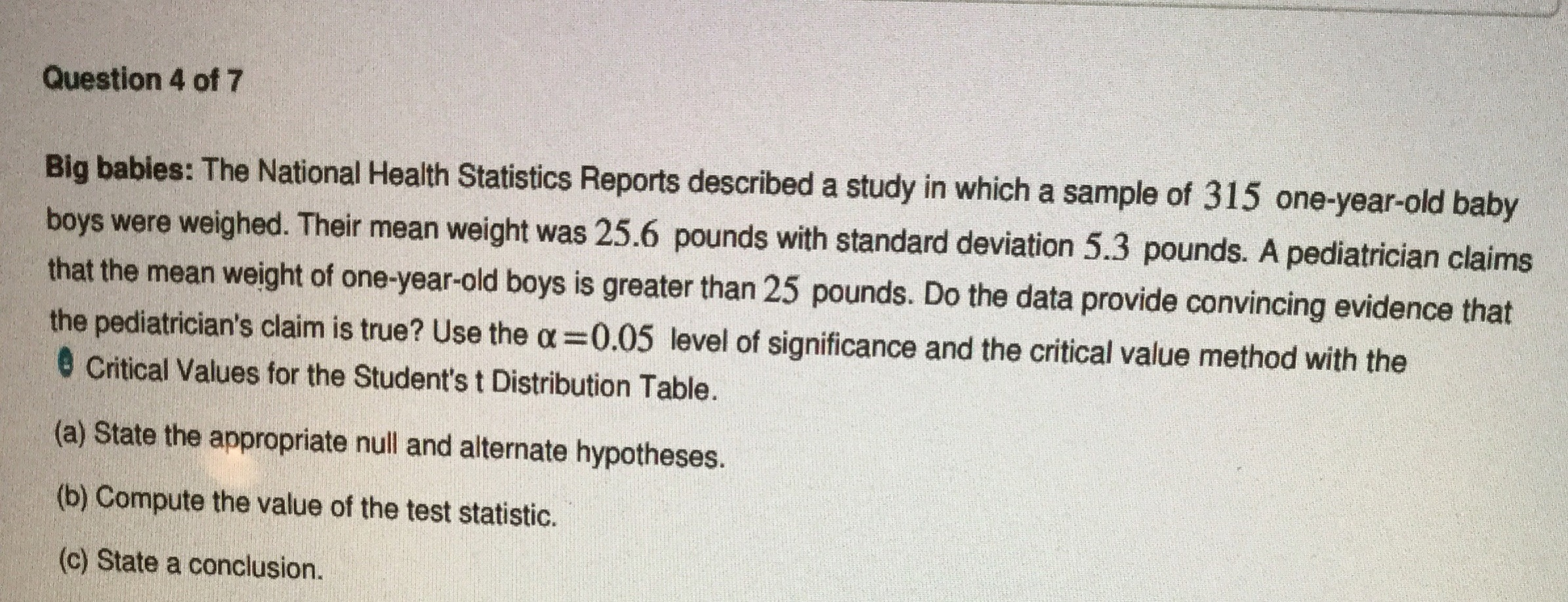# Question 4 of 7Big babies: The National Health Statistics Reports described a study in which a sample of 315 one-year-old babyboys were weighed. Their mean weight was 25.6 pounds with standard deviation 5.3 pounds. A pediatrician claimsthat the mean weight of one-year-old boys is greater than 25 pounds. Do the data provide convincing evidence thatthe pediatrician's claim is true? Use the o 0.05 level of significance and the critical value method with the@ Critical Values for the Student's t Distribution Table.(a) State the appropriate null and alternate hypotheses.(b) Compute the value of the test statistic.(c) State a conclusion.

Questionhelp_outlineImage TranscriptioncloseQuestion 4 of 7 Big babies: The National Health Statistics Reports described a study in which a sample of 315 one-year-old baby boys were weighed. Their mean weight was 25.6 pounds with standard deviation 5.3 pounds. A pediatrician claims that the mean weight of one-year-old boys is greater than 25 pounds. Do the data provide convincing evidence that the pediatrician's claim is true? Use the o 0.05 level of significance and the critical value method with the @ Critical Values for the Student's t Distribution Table. (a) State the appropriate null and alternate hypotheses. (b) Compute the value of the test statistic. (c) State a conclusion. fullscreen
check_circleExpert Solution
Step 1

From the given information,

Sample size (n) =315, mean (μ) =25.6, standard deviation (σ) =5.3 and level of significance (α) =0.05.

Step 2

(a) Null and alternative hypotheses are

H0: μ>25 (A pediatrician claims that the mean weight of one-year old boys is greater than 25 pounds).

Ha: μ≤25 (A pediatrician claims that the mean weight of one-year old boys is less than or equal to 25

Pounds (or not greater than 25)).

Step 3

(b) Now, test sta...

### Want to see the full answer?

See Solution

#### Want to see this answer and more?

Solutions are written by subject experts who are available 24/7. Questions are typically answered within 1 hour*

See Solution
*Response times may vary by subject and question
Tagged in

### Statistics